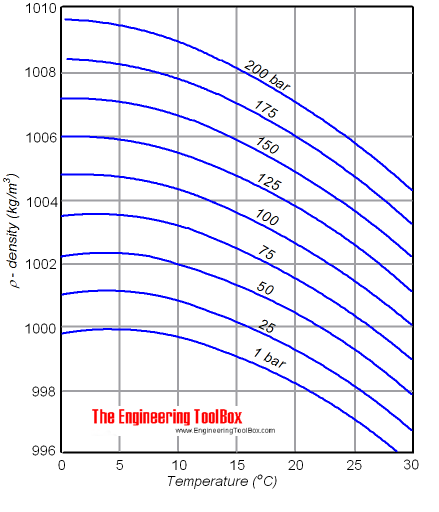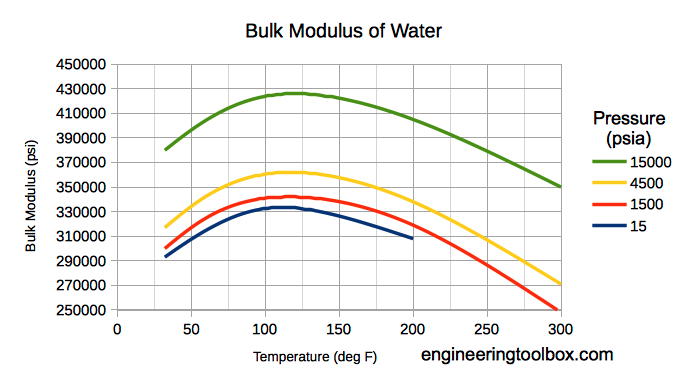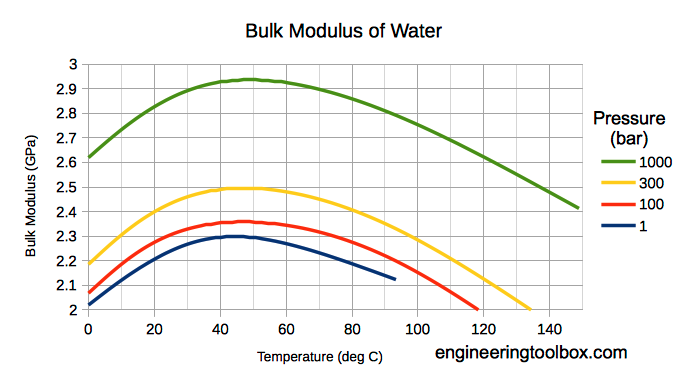Engineering ToolBox - Resources, Tools and Basic Information for Engineering and Design of Technical Applications!

# Liquids - Densities vs. Pressure and Temperature Change

## Densities and specific volume of liquids vs. pressure and temperature change.

The density of a liquid will change with temperature and pressure. The density of water versus temperature and pressure is indicated below:See also Water - Density, Specific Weight and Thermal Expantion Coefficient, for online calculator, figures and tables showing changes with temperature.

### Density

The density of a liquid can be expressed as

ρ = m / V                                (1)

where

ρ = density of liquid (kg/m3)

m = mass of the liquid (kg)

V = volume of the liquid (m3)

The inverse of density is specific volume:

v = 1 / ρ

= V / m                             (2)

where

v = specific volume (m3/kg)

### Volume and change in Temperature

When temperature increases -  most liquids expands:

dV = V1 - V0

= V0 β dt

= V0 β (t1 - t0)                               (3)

where

dV = V1 - V0 = change in volume - difference between final and initial volume (m3)

β = volumetric temperature expansion coefficient (m3/m3 oC)

dt = t1 - t0 = change in temperature - difference between final and initial temperature (oC)

(3) can be modified to

V1 = V0 (1 + β (t1 - t0))                           (3b)

### Density and change in Temperature

With (1) and (3b) the final density after a temperature change can be expressed as

ρ1 = m / (V0 (1 + β (t1 - t0)))                              (4)

where

ρ1 = final density (kg/m3)

- or combined with (2)

ρ1ρ0 / (1 + β (t1 - t0))                                  (4b)

where

ρ0 = initial density (kg/m3)

#### Volumetric Temperature Coefficients - β

Note! - volumetric temperature coefficients may vary strongly with temperature.

### Density and change in Pressure

The influence of pressure on the volume of a liquid can be expressed with the three dimensional Hooke's law

E = - dp / (dV / V0

= - (p1 - p0) / ((V1 - V0) / V0)                                   (5)

where

E = bulk modulus - liquid elasticity (N/m2)

The minus sign corresponds to the fact that an increase in the pressure leads to a decrease in volume.

With (5) - the final volume after pressure change can be expressed as

V1 = V0 (1 - (p1 - p0) / E)                                     (5b)

Combining (5b) with (1) - the final density can be expressed as:

ρ1 = m / (V0 (1 - (p1 - p0) / E))                                  (6)

- or combined with (2) - the final density can be expressed as

ρ1 = ρ0 / (1 - (p1 - p0) / E)                                  (6b)

#### Bulk Modulus Fluid Elasticity some common Fluids - E

• water : 2.15 109 (N/m2)
• ethyl alcohol : 1.06 109 (N/m2)
• oil : 1.5 109 (N/m2)

Note! Bulk modulus for liquids varies with pressure and temperature.

#### Bulk modulus for water - Imperial Units#### Bulk modulus for Water - SI units### Density of a fluid changing both Temperature and Pressure

The density of a fluid when changing both temperature and pressure can be expressed combining (4b) and (6b):

ρ1ρ1(from eq.1) / (1 - (p1 - p0) / E)

= ρ0 / (1 + β (t1 - t0)) / (1 - (p1 - p0) / E)                                (7)

### Example - Density of Water at 100 bar and 20oC

• density of water 0oC: 999.8 (kg/m3)
• expansion coefficient of water at 10oC: 0.000088 (m3/m3oC) (average value between 0 and 20oC)
• bulk modulus of water: 2.15 10(N/m2)

Density of water can be calculated with (3):

ρ1 = (999.8 kg/m3) / (1 + (0.000088 m3/m3oC) ((20 oC) - (0 oC))) / (1 - ((100 105 Pa) - (1 105 Pa)) / (2.15 109 N/m2)

= 1002.7 (kg/m3)

## Related Topics

• ### Densities

Densities of solids, liquids and gases. Definitions and convertion calculators.
• ### Fluid Mechanics

The study of fluids - liquids and gases. Involving velocity, pressure, density and temperature as functions of space and time.
• ### Thermodynamics

Work, heat and energy systems.

## Related Documents

• ### Alcohols and Carboxylic Acids - Physical Data

Molweight, melting and boiling point, density, pKa-values, as well as number of carbon and hydrogen atoms in molecules are given for 150 different alcohols and acids.
• ### Bulk Modulus and Fluid Elasticities

Introduction to - and definition of - Bulk Modulus Elasticity commonly used to characterize the compressibility of fluids.
• ### Crude Oil - Density vs. Temperature

Variations in crude oil density are shown as function of temperatur, together with volume correction factors.
• ### Densities of Aqueous Solutions of Inorganic Chlorides

Changes in density of aqueous solutions with changes in concentration at 20°C. Density of inorganic chlorides in water is plotted as function of wt%, mol/kg water and mol/l solution.
• ### Densities of Aqueous Solutions of Inorganic Potassium Salts

Changes in density of aqueous solutions with changes in concentration at 20°C. Density of potassium salts in water is plotted as function of wt%, mol/kg water and mol/l solution.
• ### Densities of Aqueous Solutions of Inorganic Sodium Salts

Changes in density of aqueous solutions with changes in concentration at 20°C. Density of inorganic sodium salts in water is plotted as function of wt%, mol/kg water and mol/l solution.
• ### Densities of Aqueous Solutions of Organic Acids

Changes in density of aqueous solutions with changes in concentration at 20°C. Density of acetic acid, citric acid, formic acid, D-lactic acid, oxalic acid and trichloroacetic acid in water is plotted as function of wt%, mol/kg water and mol/l solution.
• ### Densities of common Products

Densities of common products - Imperial and SI-units.
• ### Density Converter

Online density converter with commonly used units.
• ### Density of Aqueous Solutions of Organic Substances as Sugars and Alcohols

Changes in density of aqueous solutions with changes in concentration at 20°C. Density of some sugars, alcohols and other organic substances in water is plotted as function of wt%, mol/kg water and mol/l solution.
• ### Density of Aqueous Solutions of some Inorganic Substances

Changes in density of aqueous solutions with changes in concentration at 20°C. Density of inorganic substances in water is plotted as function of wt%, mol/kg water and mol/l solution.
• ### Density vs. Specific Weight and Specific Gravity

An introduction to density, specific weight and specific gravity.
• ### Fuel Oils - Densities vs. Temperature

Variations in fuel oils density as function of temperatur, together with volume correction factors.
• ### Hydrocarbons - Physical Data

Molweight, melting and boiling point, density, flash point and autoignition temperature, as well as number of carbon and hydrogen atoms in each molecule for 200 different hydrocarbons.
• ### Hydrocarbons, Linear Alcohols and Acids - Densities

Density of hydrocarbons like alcohols and acids as function of carbon number at 20°C / 68°.
• ### Ice and Water - Melting Points vs. Pressure

Online calculator, figures and tables with melting points of ice to water at pressures ranging from 0 to 29000 psia (0 to 2000 bara). Temperature given as °C, °F, K and °R.
• ### Jet Fuel - Density vs. Temperature

Variations in jet fuel density as function of temperatur, together with volume correction factors.
• ### Liquids - Densities

Densities of common liquids like acetone, beer, oil, water and more.
• ### Liquids - Volumetric Expansion Coefficients

Volumetric - or cubical - expansion coefficients for common liquids.
• ### Lubricating Oil - Densities vs. Temperature

Variations in lubricating oil density as function of temperatur, together with volume correction factors.
• ### Minerals - Densities

Densities of minerals.
• ### Organic Sulfur Compounds - Densities

Liquid density of different kinds of organic sulfur compounds with varying carbon number (20°C/68°F). Comparison of thiols, sulfides, disulfides and thiophenes.
• ### Volumetric (Cubic) Thermal Expansion

Volumetric temperature expansion calculator.
• ### Water - Density, Specific Weight and Thermal Expansion Coefficients

Definitions, online calculator and figures and tables with water properties like density, specific weight and thermal expansion coefficient of liquid water at temperatures ranging 0 to 360°C (32 to 680°F).
• ### Water - Saturation Pressure vs. Temperature

Online calculator, figures and tables with water saturation (vapor) pressure at temperatures ranging 0 to 370 °C (32 to 700°F) - in Imperial and SI Units.
• ### Water - Thermophysical Properties

Thermal properties of water at different temperatures like density, freezing temperature, boiling temperature, latent heat of melting, latent heat of evaporation, critical temperature and more.

## Engineering ToolBox - SketchUp Extension - Online 3D modeling!

Add standard and customized parametric components - like flange beams, lumbers, piping, stairs and more - to your Sketchup model with the Engineering ToolBox - SketchUp Extension - enabled for use with older versions of the amazing SketchUp Make and the newer "up to date" SketchUp Pro . Add the Engineering ToolBox extension to your SketchUp Make/Pro from the Extension Warehouse !

We don't collect information from our users. More about

## Citation

• The Engineering ToolBox (2009). Liquids - Densities vs. Pressure and Temperature Change. [online] Available at: https://www.engineeringtoolbox.com/fluid-density-temperature-pressure-d_309.html [Accessed Day Month Year].

Modify the access date according your visit.

10.2.10